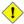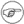# 8.1. Operators

assignment

variable assignment

Initializing or changing the value of a variable

=

All-purpose assignment operator, which works for both arithmetic and string assignments.

 ``` 1 var=27 2 category=minerals # No spaces allowed after the "=".```Do not confuse the "=" assignment operator with the = test operator.

 ``` 1 # = as a test operator 2  3 if [ "\$string1" = "\$string2" ] 4 # if [ "X\$string1" = "X\$string2" ] is safer, 5 # to prevent an error message should one of the variables be empty. 6 # (The prepended "X" characters cancel out.) 7 then 8  command 9 fi```

arithmetic operators

+

plus

-

minus

*

multiplication

/

division

**

exponentiation
 ``` 1 # Bash, version 2.02, introduced the "**" exponentiation operator. 2  3 let "z=5**3" 4 echo "z = \$z" # z = 125```

%

modulo, or mod (returns the remainder of an integer division operation)

 ``` bash\$ echo `expr 5 % 3` 2 ```

This operator finds use in, among other things, generating numbers within a specific range (see Example 9-23 and Example 9-26) and formatting program output (see Example 26-11 and Example A-7). It can even be used to generate prime numbers, (see Example A-17). Modulo turns up surprisingly often in various numerical recipes.

Example 8-1. Greatest common divisor

 ``` 1 #!/bin/bash 2 # gcd.sh: greatest common divisor 3 # Uses Euclid's algorithm 4  5 # The "greatest common divisor" (gcd) of two integers 6 #+ is the largest integer that will divide both, leaving no remainder. 7  8 # Euclid's algorithm uses successive division. 9 # In each pass, 10 #+ dividend <--- divisor 11 #+ divisor <--- remainder 12 #+ until remainder = 0. 13 #+ The gcd = dividend, on the final pass. 14 # 15 # For an excellent discussion of Euclid's algorithm, see 16 # Jim Loy's site, http://www.jimloy.com/number/euclids.htm. 17  18  19 # ------------------------------------------------------ 20 # Argument check 21 ARGS=2 22 E_BADARGS=65 23  24 if [ \$# -ne "\$ARGS" ] 25 then 26  echo "Usage: `basename \$0` first-number second-number" 27  exit \$E_BADARGS 28 fi 29 # ------------------------------------------------------ 30  31  32 gcd () 33 { 34  35  # Arbitrary assignment. 36  dividend=\$1 # It does not matter 37  divisor=\$2 #+ which of the two is larger. 38  # Why? 39  40  remainder=1 # If uninitialized variable used in loop, 41  #+ it results in an error message 42  #+ on first pass through loop. 43  44  until [ "\$remainder" -eq 0 ] 45  do 46  let "remainder = \$dividend % \$divisor" 47  dividend=\$divisor # Now repeat with 2 smallest numbers. 48  divisor=\$remainder 49  done # Euclid's algorithm 50  51 } # Last \$dividend is the gcd. 52  53  54 gcd \$1 \$2 55  56 echo; echo "GCD of \$1 and \$2 = \$dividend"; echo 57  58  59 # Exercise : 60 # -------- 61 # Check command-line arguments to make sure they are integers, 62 #+ and exit the script with an appropriate error message if not. 63  64 exit 0```

+=

"plus-equal" (increment variable by a constant)

let "var += 5" results in var being incremented by 5.

-=

"minus-equal" (decrement variable by a constant)

*=

"times-equal" (multiply variable by a constant)

let "var *= 4" results in var being multiplied by 4.

/=

"slash-equal" (divide variable by a constant)

%=

"mod-equal" (remainder of dividing variable by a constant)

Arithmetic operators often occur in an expr or let expression.

Example 8-2. Using Arithmetic Operations

 ``` 1 #!/bin/bash 2 # Counting to 6 in 5 different ways. 3  4 n=1; echo -n "\$n " 5  6 let "n = \$n + 1" # let "n = n + 1" also works. 7 echo -n "\$n " 8  9 : \$((n = \$n + 1)) 10 # ":" necessary because otherwise Bash attempts 11 #+ to interpret "\$((n = \$n + 1))" as a command. 12 echo -n "\$n " 13  14 n=\$((\$n + 1)) 15 echo -n "\$n " 16  17 : \$[ n = \$n + 1 ] 18 # ":" necessary because otherwise Bash attempts 19 #+ to interpret "\$[ n = \$n + 1 ]" as a command. 20 # Works even if "n" was initialized as a string. 21 echo -n "\$n " 22  23 n=\$[ \$n + 1 ] 24 # Works even if "n" was initialized as a string. 25 #* Avoid this type of construct, since it is obsolete and nonportable. 26 echo -n "\$n "; echo 27  28 # Thanks, Stephane Chazelas. 29  30 exit 0```Integer variables in Bash are actually signed long (32-bit) integers, in the range of -2147483648 to 2147483647. An operation that takes a variable outside these limits will give an erroneous result.
 ``` 1 a=2147483646 2 echo "a = \$a" # a = 2147483646 3 let "a+=1" # Increment "a". 4 echo "a = \$a" # a = 2147483647 5 let "a+=1" # increment "a" again, past the limit. 6 echo "a = \$a" # a = -2147483648 7  # ERROR (out of range)```Bash does not understand floating point arithmetic. It treats numbers containing a decimal point as strings.
 ``` 1 a=1.5 2  3 let "b = \$a + 1.3" # Error. 4 # t2.sh: let: b = 1.5 + 1.3: syntax error in expression (error token is ".5 + 1.3") 5  6 echo "b = \$b" # b=1```
Use bc in scripts that that need floating point calculations or math library functions.

bitwise operators. The bitwise operators seldom make an appearance in shell scripts. Their chief use seems to be manipulating and testing values read from ports or sockets. "Bit flipping" is more relevant to compiled languages, such as C and C++, which run fast enough to permit its use on the fly.

bitwise operators

<<

bitwise left shift (multiplies by 2 for each shift position)

<<=

"left-shift-equal"

let "var <<= 2" results in var left-shifted 2 bits (multiplied by 4)

>>

bitwise right shift (divides by 2 for each shift position)

>>=

"right-shift-equal" (inverse of <<=)

&

bitwise and

&=

"bitwise and-equal"

|

bitwise OR

|=

"bitwise OR-equal"

~

bitwise negate

!

bitwise NOT

^

bitwise XOR

^=

"bitwise XOR-equal"

logical operators

&&

and (logical)

 ``` 1 if [ \$condition1 ] && [ \$condition2 ] 2 # Same as: if [ \$condition1 -a \$condition2 ] 3 # Returns true if both condition1 and condition2 hold true... 4  5 if [[ \$condition1 && \$condition2 ]] # Also works. 6 # Note that && operator not permitted within [ ... ] construct.```&& may also, depending on context, be used in an and list to concatenate commands.
||

or (logical)

 ``` 1 if [ \$condition1 ] || [ \$condition2 ] 2 # Same as: if [ \$condition1 -o \$condition2 ] 3 # Returns true if either condition1 or condition2 holds true... 4  5 if [[ \$condition1 || \$condition2 ]] # Also works. 6 # Note that || operator not permitted within [ ... ] construct.```Bash tests the exit status of each statement linked with a logical operator.

Example 8-3. Compound Condition Tests Using && and ||

 ``` 1 #!/bin/bash 2  3 a=24 4 b=47 5  6 if [ "\$a" -eq 24 ] && [ "\$b" -eq 47 ] 7 then 8  echo "Test #1 succeeds." 9 else 10  echo "Test #1 fails." 11 fi 12  13 # ERROR: if [ "\$a" -eq 24 && "\$b" -eq 47 ] 14 # attempts to execute ' [ "\$a" -eq 24 ' 15 # and fails to finding matching ']'. 16 # 17 # if [[ \$a -eq 24 && \$b -eq 24 ]] works 18 # (The "&&" has a different meaning in line 17 than in line 6.) 19 # Thanks, Stephane Chazelas. 20  21  22 if [ "\$a" -eq 98 ] || [ "\$b" -eq 47 ] 23 then 24  echo "Test #2 succeeds." 25 else 26  echo "Test #2 fails." 27 fi 28  29  30 # The -a and -o options provide 31 #+ an alternative compound condition test. 32 # Thanks to Patrick Callahan for pointing this out. 33  34  35 if [ "\$a" -eq 24 -a "\$b" -eq 47 ] 36 then 37  echo "Test #3 succeeds." 38 else 39  echo "Test #3 fails." 40 fi 41  42  43 if [ "\$a" -eq 98 -o "\$b" -eq 47 ] 44 then 45  echo "Test #4 succeeds." 46 else 47  echo "Test #4 fails." 48 fi 49  50  51 a=rhino 52 b=crocodile 53 if [ "\$a" = rhino ] && [ "\$b" = crocodile ] 54 then 55  echo "Test #5 succeeds." 56 else 57  echo "Test #5 fails." 58 fi 59  60 exit 0```

The && and || operators also find use in an arithmetic context.

 ``` bash\$ echo \$(( 1 && 2 )) \$((3 && 0)) \$((4 || 0)) \$((0 || 0)) 1 0 1 0 ```

miscellaneous operators

,

comma operator

The comma operator chains together two or more arithmetic operations. All the operations are evaluated (with possible side effects), but only the last operation is returned.

 ``` 1 let "t1 = ((5 + 3, 7 - 1, 15 - 4))" 2 echo "t1 = \$t1" # t1 = 11 3  4 let "t2 = ((a = 9, 15 / 3))" # Set "a" and calculate "t2". 5 echo "t2 = \$t2 a = \$a" # t2 = 5 a = 9```

The comma operator finds use mainly in for loops. See Example 10-12.